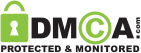Medical

Are you Overweight, Underweight, Obese or at a Normal Weight?

We always need to monitor our weight to make sure that we are healthy or that we are overweight and need to lose weight through changing our bad habits in eating or through doing exercises. The most common way for showing you this is using what is called BMI. BMI is an acronym for Body Mass Index and it is a measure that is used for deciding the shape of our bodies. Through using BMI, you will be able to determine whether you are at a normal weight, overweight, underweight or you suffer from obesity. Body mass index (BMI) is based on two main things which are your height and your mass or weight. In order to be able to calculate your BMI on your own, follow these steps.

♦ Find your weight: Use your bathroom scale to weigh yourself and read it in pounds because our formula will be based on this.♦ Get your height: Use a measuring tape or a yardstick to measure your height and get it in inches not in centimeters for the formula that we will use. You can ask someone to measure your height or you can do it on your own through standing against a wall and marking the top of your head with a pencil to measure it.The formula of the BMI is:

BMI = mass (kg) / ( height (m))

or

BMI = ( mass (lb) / ( height(in))) x 703 → The formula that we will use.

♦ Square the number of your height that you have taken before in inches: It means to multiply the number by itself or by the same number.

♦ Divide the number of your weight: Take the number of your weight in pounds and divide it by the number of your height in inches that you squared before.

♦ Use the conversion factor: Multiply the value that you get from the former step by the number 703 which is the conversion factor. The number 703 is only used when you take your height and weight in inches and pounds , but when you take them in centimeters and kilograms, then skip using the number 703.

♦ Use the BMI scale to judge your BMI: After getting the value of your BMI, judge it using the following scale.► BMI that is of or less than 18.5 shows that you are underweight.

BMI = < 18.5 → underweight

► BMI that ranges from 18.6 to 24.9 shows that you are at a normal weight.

BMI = 18.6 – 24.9 → normal weight

► BMI that ranges from 25 to 29.9 shows that you are overweight.

BMI = 25 – 29.9 → overweight

► BMI that is of 30 or more shows that you are obese.

BMI = > 30 → obesity

Example

If there is a person whose weight is 65 kg and the height is 1.8 cm, then the BMI is

BMI = 65 / (1.8)= 65 / 3.24 = 20.06= 20.1 which means that this person is at a normal weight according to the scale.

The other formula which uses pounds and inches

To get the BMI for the same person that we get before, we have to convert the previous measurements.

1.8 m → 70.9 in

65 kg → 143 lbs

BMI = (143 / (70.9)2 ) x 703 = (143 / 5026.81) x 703 = 19.9 = 20

Now, start calculating your BMI on your own to know whether you are overweight, underweight, obese or at a normal weight.Close

Pin It on PinterestClose